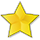BrainDen.com - Brain Teasers

Question

I find probability questions interesting, because they often defy intuition. Particularly for me are those that involve waiting times. Other than the basic idea of an event of probability p needing on average 1/p trials to occur. But here's one not that trivial, yet still fairly easy to solve -- with the right approach.

On average, how many times do you need to flip a fair coin before you have seen a (continuous) run of an odd number of heads followed by a tail?

For example, T T H H H H T H H H T took 11 flips.

Edited by bonanova
clarification

Recommended Posts

• 0
Spoiler

As you said, it IS easy, after a while of thinking I’m clueless.

My solution is a recursive evaluation, based on a two/state machine.
The two states are based on parity of H seen so far, “Even” and “odd”

In even state

H -> 1/2 (1+ odd)
T -> 1/2 (1+ even)

To summarise:

Even = 1/2(1+odd) + 1/2(1+even)

Similarly,

Odd = 1/2(1+even) + 1/2 = 1 + even /2

Substitute odd into even

Even = 1/2 + 1/2(1+even/2) + 1/2 +even/2
= 3/2  + 3even/4

Solve for even

1/4 even = 3/2
Even =6

Edited by bonanova
Share on other sites

• 0
6 hours ago, CaptainEd said:
Hide contents

... based on parity of H seen so far  ...

Not sure if it was clear that the run of odd number of heads are contiguous, as in the example, or if I'm misunderstanding your algorithm. Can you add some words here and there?

Share on other sites

• 0
Spoiler

Before any coin is flipped, there are an even number of heads. This is the Even state; if a Head is flipped, we go to the Odd state. Whenever we flip a Tail in Even state, we are back to zero which is Even state. If we get a Tail in Odd state, we win!

If we get a Head in Odd state, we go to Even state. If we get a tail in Even state, we go to Odd state.

Edited by bonanova
Added spoiler / reduced type size
Share on other sites

• 0

Transition diagram

In Even State: H->Odd, T-> Even

in Odd state: H-> Even, T->Terminate(win)

i believe this is sufficient to keep track of parity of Heads.

each transition adds 1 to Flip count

Edited by CaptainEd
Share on other sites

• 0

@CaptainEd - Nice solve.Here is a solution i was aware of.
I think the two are similar or equivalent, parsed out into a different set of states.

Spoiler

Let e be the expected number of flips.
There are three early states that are easy to analyze and cover all the possibilities:

1. T consumes 1 flip and, because it cannot be part of the solution, requires e more flips.
2. HH consumes 2 flips and, even tho it could be part of a solution, it also requires e more flips.
3. HT consumes 2 flips and, because it is a solution, requires no more flips.

That allows us to write an expression for x as the sum of these terms, weighted by their respective probabilities: 1/2, 1/4, 1/4.

e = 1/2{1+e} + 1/4{2+e} + 1/4{2}.

4e = 2+2e + 2+e + 2.

e = 6

Share on other sites

• 0

much more clearly stated than my babbling. I’m tickled that I’ve shown that it can be evaluated one flip at a time, based merely on the parity of contiguous Hs.

here is my argument, expressed by plagiarizing your expression:

Let e be the expected number of flips from the initial (even) state
There are two states that are easy to analyze and cover all the possibilities:

1. E is the initial state, it also represents the state of having seen an even number of H (including zero), since the beginning or the most recent T.
2. O is the odd state, representing the fact of having seen an odd contiguous run of H.
3. State E  requires e more flips. In this state, H changes to state O, while T remains in state E
4. State O requires more flips. In this state, H changes to state E, while T terminates with a win.

That allows us to write an expression for x as the sum of these terms, weighted by their respective probabilities, all 1/2.

e = 1/2{1+e} + 1/2{1+o}

o = 1/2{1+e} + 1/2

substitute o into e

e = 1/2{1+e} + 1/2{1+1/2{1+e} + 1/2}

1/2{1+e} + 1/2+1/4+e/4 + 1/4

= 3/2 + 3e/4

e/4 = 3/2

e = 6

Join the conversation

You can post now and register later. If you have an account, sign in now to post with your account.×   Pasted as rich text.   Paste as plain text instead

Only 75 emoji are allowed.

×   Your previous content has been restored.   Clear editor

×   You cannot paste images directly. Upload or insert images from URL.